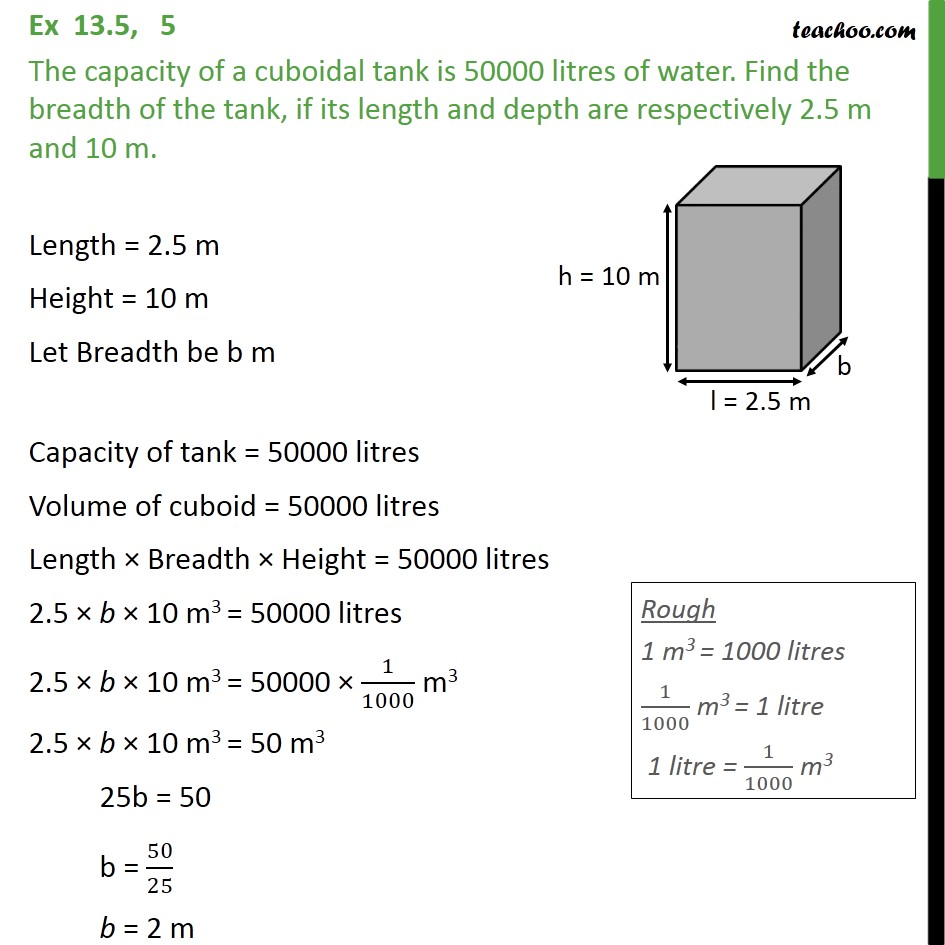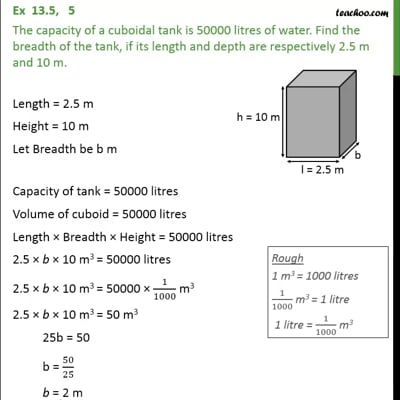Ex 13.5

Chapter 13 Class 9 Surface Areas and Volumes (Term 2)
Serial order wiseThis video is only available for Teachoo black users

### Transcript

Ex 13.5, 5 The capacity of a cuboidal tank is 50000 litres of water. Find the breadth of the tank, if its length and depth are respectively 2.5 m and 10 m. Length = 2.5 m Height = 10 m Let Breadth be b m Capacity of tank = 50000 litres Volume of cuboid = 50000 litres Length × Breadth × Height = 50000 litres 2.5 × b × 10 m3 = 50000 litres 2.5 × b × 10 m3 = 50000 × 1/1000 m3 2.5 × b × 10 m3 = 50 m3 25b = 50 b = 50/25 b = 2 m